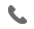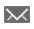•+44 7459 302492info@uplatz.com
• Register
Job Meter = High

### Introduction to Applied Econometrics

30 Hours
Online Instructor-led Training
USD 2800318 Learners

This course, the first of two, focuses on the development and use of single-equation econometric models that enable analysts to better understand their economic/business landscape and to improve their ability to make sound economic/business forecasts. Through hands-on exercises, participants gain knowledge of the practical elements of applied econometric analysis. The overall aims are to sharpen the quantitative, statistical, and analytical skills of participants in dealing with problems and issues related to business and economics as well as to improve communication skills in reporting findings to decision makers.

Learn how to
• Develop and use single-equation econometric models.
• Improve your ability to make sound forecasts.
• Sharpen your quantitative, statistical, and analytical skills.
• Improve the effectiveness of how you translate technical information for key decision-makers.
• Use SAS software to conduct econometric analyses.
---------------------------------------------------------
Target Audience

---------------------------------------------------------

# Introduction to Applied Econometrics

Course Details & Curriculum
The Nature of Applied Econometrics
• What is applied econometrics?
• Course of action - development of formal quantitative models.
• Disciplines in applied econometrics.
• Empirical models and modeling approaches.
• Components of applied econometrics.
• Products of applied econometrics.
• Getting started.
• Generic Multiple Regression model.
• Software considerations.
• Communication and aims for the analyst.
Data Considerations and Ordinary Least Squares Estimation of Single-Equation Econometric Models
• Data.
• Getting a feel for the data.
• Massaging the data.
• Estimation of the Simple Linear Regression model.
• SAS output of the simple linear regression of total personal bankruptcy (TPB) on real gross domestic product (RGDP).
• Estimation of the Multiple Regression model - the Generic Single-Equation Econometric model.
• Example: SAS output of the demand function for shrimp.
Interpretation and Use of Estimated Coefficients and Forecasting with Single-Equation Econometric Models
• Mathematical and statistical considerations in applied econometrics.
• Interpretation of estimated coefficients.
• Partial correlation coefficients.
• Alternatives to least squares estimation.
• Criteria for estimators.
• Interval estimation and confidence intervals.
• Forecasting with single-equation econometric models.
• Forecast evaluation.
• Illustration of out-of-sample forecasting with the demand curve for shrimp.
Common Tests of Hypotheses in Applied Econometrics
• Introduction: preliminary statistical elements.
• Basics of hypothesis testing.
• Tests of hypotheses regarding structural parameters of econometric models.
• Tests of normality of residuals.
• Tests of hypotheses associated with the specification of econometric models.
Use of Dummy (Indicator) Variables in Applied Econometrics
• Intercept shifters.
• Slope shifters.
• Intercept shifters and slope shifters.
• Final thoughts about the use of dummy (indicator) variables.
Diagnostic Checks - Autocorrelation or Serial Correlation
• Autocorrelation or serial correlation.
• Tests for serial correlation.
• Sample problem: the demand for shrimp.
• Sample problem: the demand for gasoline.
• A test for serial correlation in the presence of a lagged dependent variable.
• Summary remarks about the issue of serial correlation.
Diagnostic Checks - Heteroscedasticity
• Weighted least squares (WLS).
• Example of econometric analysis with heteroscedasticity.